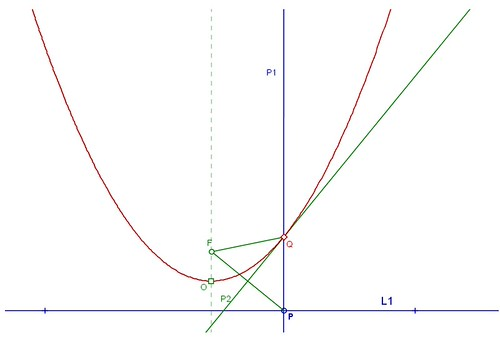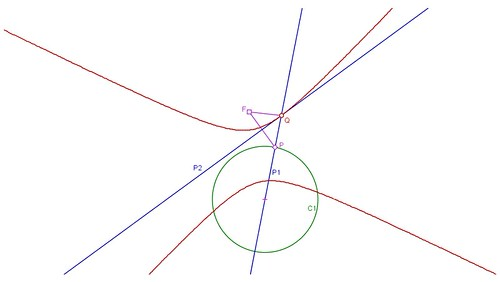## Fancies of the parabola and hyperbolaMany revolutions-of-the-sun ago we were seeking a device that could construct a parabola. We made a discovery in this connection that is exceedingly elementary Euclid for mathematicians, but for us it was an insight-giving philosophical revelation. A parabola can be made thus:1) Draw a line L1;
2) Take a point P on it;
3) Draw perpendicular line P1 through P;
4) Take a random fixed point F, which will be the focus of the future parabola;
5) Draw the segment FP;
6) Draw the perpendicular bisector line P2 of segment FP;
7) Mark the point Q where P2 intersects P1;
8) Track the locus of Q as P moves on P1, i.e. the locus of the intersection of the perpendicular bisector P2 of FP with P1 as P moves on L1;
This locus is a parabola with focus at F. All the lines P2 are tangents of this parabola.

We can obtain the equation of the parabola thus:
Place origin O (0,0) at the minimum of the parabola;
Then the focus F is at (0,a);
Because P2 is the perpendicular bisector of FP we can show that the equation of line L1 is y=-a using similarity of triangles;
Coordinates of the point on the generated locus are Q(x,y);
Hence, P(x,-a);
By bhuja-koTi-karNa-nyAya: FQ^2=PQ^2; hence, (x-0)^2+(y-a)^2=(x-x)^2+(y+a)^2;
Thus we have y=x^2/(4*a) as the equation of the parabola (or proof that the locus of Q is a parabola).

We then found that if you repeat the above procedure with a circle C1 in place of line L1, and a radial line P1 in place of the P1 of the above example we get a hyperbola:This entry was posted in Life, Scientific ramblings and tagged , , , , , , . Bookmark the permalink.